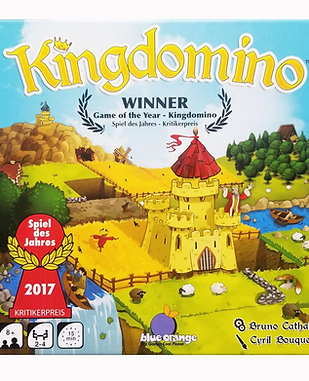top of page

# MATHLost Cities

Middle +

Addition, subtraction, multiplication, algebraic equations, risk managementBalance Beans

Preschool +

Logic, problem solving, math, early algebra, balance, simple equation, colors, counting, equalizing, and basic physics.Elementary

Addition, coin-sense, spatial reasoning, pattern recognition, early finances.Math Fluxx

Late Elementary +Kingdomino

Elementary +